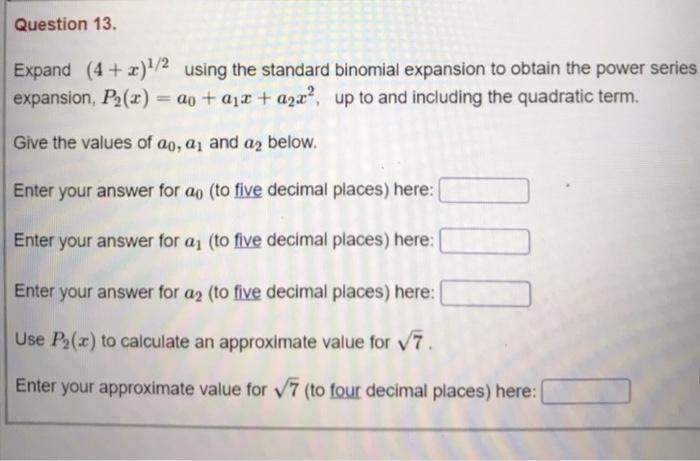### Create an Account

Already have account?

### Forgot Your Password ?

Home / Questions / Question 13. Expand (4 + x)1/2 using the standard binomial expansion to obtain the power s...

# Question 13. Expand (4 + x)1/2 using the standard binomial expansion to obtain the power series expansion, P2(x) = a0 +212 + azx?, up to and including the quadratic term. Give the values of ao, a, and

Question 13. Expand (4 + x)1/2 using the standard binomial expansion to obtain the power series expansion, P2(x) = a0 +212 + azx?, up to and including the quadratic term. Give the values of ao, a, and a2 below. Enter your answer for ao (to five decimal places) here: Enter your answer for a (to five decimal places) here: Enter your answer for a2 (to five decimal places) here: Use P(x) to calculate an approximate value for v7. Enter your approximate value for V7 (to four decimal places) here:Apr 15 2021 View more View Less

#### Answer (Solved)Subscribe To Get Solution